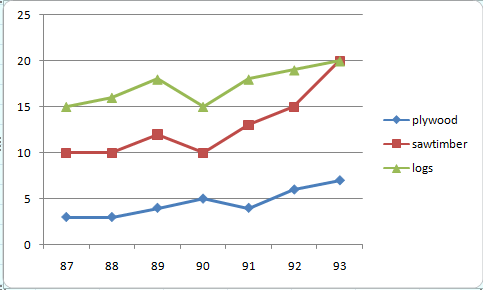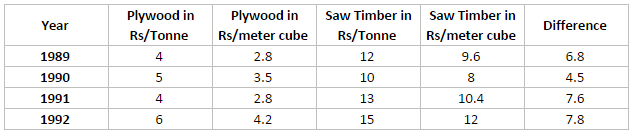# Cat Level Data Interpretation Questions With Solutions

1
5327

The data Interpretation questions in exams like CAT are in much higher or tougher level in compared to other exams likes Banking or SSC. The analysis of the given data plays much role in solving these DI problems compared to the calculation part. Here is the one solved DI set, that is asked in CAT previous paper.

Cat Level Data Interpretation Questions With Solutions:

Instructions:

Answer the questions based on the following information. In the following chart, the price of logs shown is per cubic metre that of plywood and saw timber is per tonne.Question 1:

What is the maximum percentage increase in price per cubic metre or per tonne over the previous year?

a) 33.33%

b) 85%

c) 50%

d) Cannot be determined

Question 2:

Which product shows the maximum percentage increase in price over the period?

a) Saw timber

b) Plywood

c) Logs

d) Cannot be determined

Question 3:

If the prices of sawtimber increased by 33.33%, in which year was the difference in prices of one tonne of saw timber and one cubic metre of logs the least?

a) 1989

b) 1990

c) 1991

d) 1992

Question 4:

If one cubic metre = 700 kg for plywood and 800 kg for saw timber, find in which year was the difference in the prices of plywood and saw timber (per cubic metre) the maximum?

a) 1989

b) 1990

c) 1991

d) 1992

Question 5:

If the volume sales of plywood, saw timber and logs were 40%, 30% and 30% respectively, then what was the average realisation in 1993 per cubic metre of sales? (Weight of one cubic metre of saw dust and plywood both = 800 kg)

a) 18

b) 15

c) 16

d) 13

Question 6:

In the previous question, if in 1994, prices increased by 5%, 1% and 10% while the volume sales break-up was 40%, 30% and 30% for plywood, saw timber and logs respectively, then what was the average realisation?

a) 18.95

b) 16.45

c) 13.15

d) 10.25

Solutions for CAT level Data Interpretation questions:

The maximum percentage increase in price of logs happened in the year 1991 (compared to 1990) and equaled 18/15 – 1 = 20%
The maximum percentage increase in price of plywood happened in the year 1992 (compared to 1991) and equaled 6/4 – 1 = 50%
The maximum percentage increase in price of sawtimber happened in the year 1993 (compared to 1992) and equaled 20/15 – 1 = 33.33%
Hence, the correct answer is 50%

In both imports and exports, India did the least amount of trade in 1997-98 with K (both equal to 1%).
Hence, India had the lowest total trade with K in the year 1997-98.
The amount of Indian exports equals 1%*33979 = 340 millions

After an increase by 33%, the difference in the prices of one tonne of saw timber and one cubic metre of logs is given below according to the respective years.

The difference in 1987 is 15 – 10*133.33% = 1.67
The difference in 1988 is 16 – 10*133.33% = 2.67
The difference in 1989 is 17.5 – 12*133.33% = 1.5
The difference in 1990 is 15 – 10*133.33% = 1.67
The difference in 1991 is 17.5 – 12.5*133.33% = 0.833
The difference in 1992 is 18 – 15*133.33% = -2
The difference in 1993 is 20 – 20*133.33% = -6.67
Hence, the difference is least in the year 1991.From the above diagram, we can say that difference is highest in 1992.

In 1993, price of logs = Rs. 20 per cubic metre.Price of plywood = 7/1.33 = Rs 5.26 per cubic metre. Price of saw timber = 19/1.33 = Rs 14.28 per cubic metre.
We know that the sales volume of plywood, saw timber and logs are in the ratio 4 : 3 : 3.
Thus, the average realization must be the weighted avg of these three-
$$\frac{(4\times 5.26)+(3 \times 14.28)+(3 \times 20)}{4+3+3}$$
=12.4
= Rs. 13 (Approximately)

In this case the average realization will be-

$$\frac{(4\times 5.26 \times 1.05)+(3 \times 14.28 \times 1.01)+(3 \times 20 \times 1.1)}{4+3+3} \approx 13.15$$

#### 1 COMMENT

1.Goutham Reddy